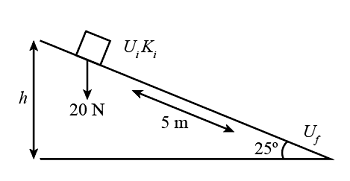# A 5.00 \ kg carrying a charge of Q=60 \ \mu C is connected to a spring for which k=100 \ N/m. The...

## Question:

A {eq}5.00 \ kg {/eq} carrying a charge of {eq}Q=60 \ \mu C {/eq} is connected to spring for which {eq}k=100 \ N/m {/eq}. The block lies on a frictionless horizontal track and the system is immersed in a uniform electric field of {eq}E=5.0e^5 \ V/m {/eq}. If the block is released from rest when the spring is unstretched.

a) By what maximum amount does the spring expand?

b) What is the equilibrium position of the block?

c) if the block is released from rest at {eq}x=0 {/eq}, how fast is it moving at {eq}x=0.25 \ m {/eq}?

## Energy lost:

The energy lost can be defined as when the body moves on surface, losses the energy in the form of heat and friction. Mathematically, the amount of energy provided to move the body will be equal to the energy lost and the work done by the body.

Given Data:

• The force of crate is, {eq}F = 20\;{\rm{N}} {/eq}
• The length of ramp is, {eq}l = 5.0\;{\rm{m}} {/eq}
• The loss in energy is, {eq}20\;{\rm{J}} {/eq}From the above figure,

{eq}\begin{align*} h &= 5\sin 25^\circ \\ h &= 2\;{\rm{m}} \end{align*} {/eq}

The initial potential energy is calculated as,

{eq}\begin{align*} {U_i} &= mgh\\ {U_i} &= \left( {\dfrac{{20}}{{9.8}}} \right) \times 9.8 \times 2\\ {U_i}& = 40\;{\rm{J}} \end{align*} {/eq}

If there is no friction then,

{eq}\begin{align*} \Delta K + \Delta U &= 0\\ \left( {{K_f} - {K_i}} \right) + \left( {{U_f} - {U_i}} \right) &= 0\\ 40 - 40& = 0 \end{align*} {/eq}

But if there is a friction and {eq}K_f^' < {K_f} {/eq}

Then,

{eq}\begin{align*} \Delta E &= \left( {K_f^' - {K_i}} \right) + \left( {{U_f} - {U_i}} \right)\\ \Delta E &= 20 - 40\\ \Delta E &= - 20\;{\rm{J}} \end{align*} {/eq}

Therefore, kinetic energy equals to,

{eq}\begin{align*} K_f' &= \dfrac{1}{2}m{v^2}\\ 20 &= \dfrac{1}{2} \times \left( {\dfrac{{20}}{{9.8}}} \right) \times {v^2}\\ v &= 4.4\;{\rm{m/s}} \end{align*} {/eq}

Thus, the speed of the crate at the bottom of the incline is {eq}4.4\;{\rm{m/s}} {/eq}.Conservation of Kinetic Energy

from

Chapter 19 / Lesson 5
31K

Kinetic energy is the energy of motion. In this lesson, we investigate how kinetic energy is sometimes conserved and sometimes not conserved, based on the type of collisions between masses.